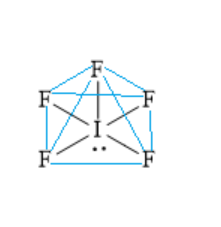Shape and hybridization of ${\text{I}}{{\text{F}}_{\text{5}}}$ respectively are ________.A ) trigonal bipyramidal, $s{p^3}d$B ) see-saw $s{p^3}d$C ) square pyramidal, $s{p^3}{d^2}$D ) pentagonal pyramidal $s{p^3}{d^3}$Verified
118.5k+ views
Hint: Determine the steric number of the molecule. For this, determine the number of bond pairs and lone pairs of electrons present on the central atom in the molecule. For this, determine the electronic configuration and the valence of the central atom.

The electronic configuration of the central iodine atom is $\left[ {{\text{Kr}}} \right]{\text{5}}{{\text{s}}^2}5{p^2}$.
There are seven valence electrons in the central iodine atom. Draw the Lewis dot structure.Out of seven valence electrons of central iodine atoms, five valence electrons are shared with electrons of five fluorine atoms to form five iodine-fluorine bonds. One lone pair of electrons remains on the central iodine atom.
Thus, for the central iodine atom, the number of bonded atoms is five and the number of lone pairs of electrons is one. The steric number is .$5 + 1 = 6$.
Steric number of six is associated with $s{p^3}{d^2}$ hybridization.
The electron pair geometry is octahedral and the molecular geometry is square pyramidal. Four fluorine atoms and one iodine atom are in one plane. One fluorine atom is present above the plane. Below the plane, one lone pair of electrons is present.
Hence, the correct option is C ) square pyramidal, $s{p^3}{d^2}$.

Additional information: Steric number five is associated with $s{p^3}d$ hybridization and trigonal bipyramidal shape, when five bond pairs of electrons and zero lone pair of electrons are present. When four bond pairs of electrons and zero lone pair of electrons are present, the shape is see-saw.
Steric number seven is associated with $s{p^3}{d^3}$ hybridization and pentagonal bipyramidal shape

Note:
Two different molecules having the same hybridization can have different geometry. This is due to the difference in the number of bond pairs and lone pairs of electrons present in two molecules having the same steric number. Steric number is the sum of the number of lone pairs of electrons and the bond pairs of electrons present in the central atom. Steric number is used to determine hybridization and molecular geometry.